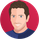Bahasa Melayu
Dilihat 127 kali
127
This is the follow up to my post on the SPX Developing Impulse Wave from 2/11/16.

The sub waves of the supposed Minute wave "IV" Running Triangle are as follows.

(a) = 43.90
(b) = 67.40
(c) = 33.80
(d) = 21.90
(e) = 17.40

The Fibonacci relationships between the waves are as follows.
67.40(b)/43.90(a) = 1.53
33.80(c)/67.40(b) = .50
17.40(e)/33.80(c) = .514
21.90(d)/43.90(a) = .498
17.40(e)/43.90(a) = .396

The Fibonacci sub wave relationships of Minute wave "IV" support the proposition that the SPX is forming an Impulse wave from 2/11/16 and that Minor wave "3" is nearing completion.

Markhi mark, thank you, the numbers @ (a) etc .... what do they stand for ? how do you calculate them ?
thank you
BalasDaxiDriver
Hi @DaxiDriver,

The numbers for each sub wave for example Minuette wave (a) are calculated by measuring the distance from the sub waves point of origin to its termination point.
The point of origin for Minuette wave (a) is the termination point of Minute wave "III" at SPX 2277.50 - 2233.60 the termination point of Minuette wave (a) = 43.90.

The terms Minute wave and Minuette wave are used to describe the degree of the Elliott waves.

Mark

Balas
MS Bahasa Melayu
EN English
EN English (UK)
EN English (IN)
DE Deutsch
FR Français
ES Español
IT Italiano
PL Polski
SV Svenska
TR Türkçe
RU Русский
PT Português
ID Bahasa Indonesia
TH ภาษาไทย
VI Tiếng Việt
JA 日本語
KO 한국어
ZH 简体中文
ZH 繁體中文
AR العربية
HE עברית